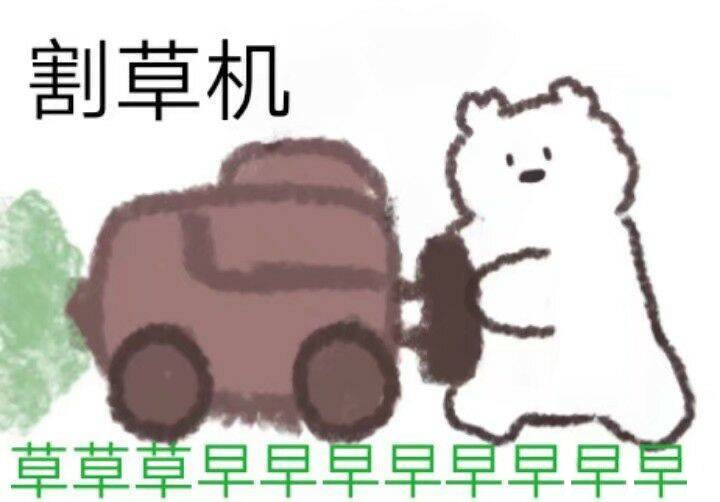# 2020 年 “联想杯”全国高校程序设计在线邀请赛暨第三届上海理工大学程序设计竞赛

H. Hay Mower
PDF 题面可用

Are you tired of city life? Have you ever had illusions of pastoral peace? The clean atmosphere, the closeness to nature and the gentle pace of living, all made Setsuna yearn for the pastoral life more.

In order to experience the simple pastoral life, Setsuna has moved to Star Valley and started her farming journey.

Soon, she discovers the problem: overgrown weeds are harming her farm. In Chinese, we call it “Sheng Cao”. She realized that weeding should be put at the top priority.The farm can be described as an $n \times m$ matrix. The growth rate of weed in row $i$ and column $j$ is denoted as $a_{i,j}$, indicating that the weed will grow $a_{i,j}$ units every beginning of the moment. At the end of moment $0$, there is no weed on the farm.

Setsuna will use mower $k$ times, where the $i$-th use occurs at the end of moment $t_i$. Each use of the mower completely removes weeds in a row or column.

Setsuna wonders how many units of weed she will remove.

The answer might be very large, so please output the desired answer modulo $998244353$.

### 输入格式

The first line contains three integers $n,m,k(1 \leq n,m \leq 500,1 \leq k \leq 3 \times 10^5)$.

The next $n$ lines contains $m$ integers each, where the $j$-th integer of the $i$-th line is $a_{i,j}(0 \leq a_{i,j} \leq 10^{18})$.

The $i$-th of the next $k$ lines contains one character and two integers.

• $r\ x_i\ t_i$ - Setsuna clears the weeds in row $x_i$ at the end of moment $t_i$.

• $c\ y_i\ t_i$ - Setsuna clears the weeds in column $y_i$ at the end of moment $t_i$.

It is guaranteed that $1 \leq x_i \leq n,1 \leq y_i \leq m,1 \leq t_i \leq 10^{18}$ hold for $1 \leq i \leq k$ and $t_i$ is strictly increasing.

### 输出格式

Output one integer indicating the answer modulo $998244353$.

### 样例

Input
2 2 3
1 2
3 4
r 1 5
c 2 6
r 1 7

Output
45

Input
3 4 1
1 2 3 4
5 6 7 8
9 10 11 12
r 1 1000000000000000000

Output
172998509


### 提示

Sample $1$:

At the end of moment $0$, the farm looks like

$$\left[ \begin{matrix} 0 & 0 \\ 0 & 0 \\ \end{matrix} \right]$$

At the end of moment $5$, Setsuna has cleared row $1$ and $15$ units of weed has been cut, so the farm looks like

$$\left[ \begin{matrix} 0 & 0 \\ 15 & 20 \\ \end{matrix} \right]$$

At the end of moment $6$, Setsuna has cleared column $2$ and $26$ units of weed has been cut, so the farm looks like

$$\left[ \begin{matrix} 1 & 0 \\ 18 & 0 \\ \end{matrix} \right]$$

At the end of moment $7$, Setsuna has cleared row $1$ and $4$ units of weed has been cut, so the farm looks like

$$\left[ \begin{matrix} 0 & 0 \\ 21 & 4 \\ \end{matrix} \right]$$

So the answer is $15+26+4=45$ units.

NaN